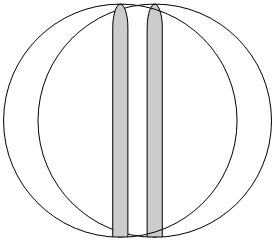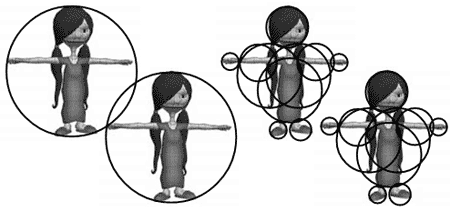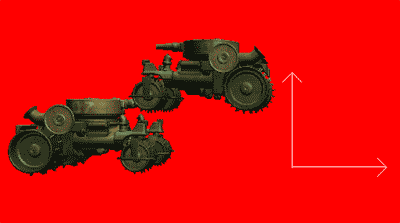# 4.10 使用包围球进行基本模型碰撞检测

## 工作原理

### 快速检测myModel = XNAUtils.LoadModelWithBoundingSphere(ref modelTransforms, "tank",Content);

private bool CoarseCheck(Model model1, Matrix world1, Model model2, Matrix world2)
{
BoundingSphere origSphere1 = (BoundingSphere)model1.Tag;
BoundingSphere sphere1= XNAUtils.TransformBoundingSphere(origSphere1, world1);

BoundingSphere origSphere2= (BoundingSphere)model2.Tag;
BoundingSphere sphere2 = XNAUtils.TransformBoundingSphere(origSphere2,world2);

bool collision = sphere1.Intersects(sphere2);
return collision;
} 

### 更加精确，但有点慢private bool FinerCheck(Model model1, Matrix world1, Model model2, Matrix world2)
{
if (CoarseCheck(model1, world1,model2, world2) == false)
return false;
bool collision = false;

Matrix[] model1Transforms= new Matrix[model1.Bones.Count];
Matrix[] model2Transforms = new Matrix[model2.Bones.Count];

model1.CopyAbsoluteBoneTransformsTo(model1Transforms);
model2.CopyAbsoluteBoneTransformsTo(model2Transforms);

foreach (ModelMesh mesh1 in model1.Meshes)
{
BoundingSphere origSphere1 = mesh1.BoundingSphere;
Matrix trans1 = model1Transforms[mesh1.ParentBone.Index] * world1;
BoundingSpheretransSphere1 = XNAUtils.TransformBoundingSphere(origSphere1, trans1);

foreach (ModelMesh mesh2 in model2.Meshes)
{
BoundingSphere origSphere2 = mesh2.BoundingSphere;
Matrix trans2 = model2Transforms[mesh2.ParentBone.Index] * world2;
BoundingSphere transSphere2 = XNAUtils.TransformBoundingSphere(origSphere2, trans2);
if (transSphere1.Intersects(transSphere2))
collision = true;
}
}
return collision;
}

### 优化

private bool FinerCheck(Model model1, Matrix world1, Model model2, Matrix world2)
{
if (CoarseCheck(model1, world1, model2, world2) == false)
return false;
Matrix[] model1Transforms = new Matrix[model1.Bones.Count];
model1.CopyAbsoluteBoneTransformsTo(model1Transforms);
BoundingSphere[] model1Spheres = new BoundingSphere[model1.Meshes.Count];

for (int i=0; i<model1.Meshes.Count; i++)
{
ModelMesh mesh = model1.Meshes[i];
BoundingSphere origSphere = mesh.BoundingSphere;
Matrix trans = model1Transforms[mesh.ParentBone.Index]* world1;
BoundingSphere transSphere = XNAUtils.TransformBoundingSphere(origSphere,trans);
model1Spheres[i] = transSphere;
}

Matrix[] model2Transforms = new Matrix[model2.Bones.Count];
model2.CopyAbsoluteBoneTransformsTo(model2Transforms);
BoundingSphere[] model2Spheres= new BoundingSphere[model2.Meshes.Count];

for (int i = 0; i < model1.Meshes.Count;i++)
{
ModelMesh mesh = model2.Meshes[i];
BoundingSphere origSphere = mesh.BoundingSphere;
Matrix trans = model2Transforms[mesh.ParentBone.Index] * world2;
BoundingSphere transSphere = XNAUtils.TransformBoundingSphere(origSphere, trans);
model2Spheres[i]= transSphere;
}

bool collision = false;
for (int i=0; i<model1Spheres.Length;i++)
for (int j = 0; j < model2Spheres.Length; j++)
if (model1Spheres[i].Intersects(model2Spheres[j]))
return true;
return collision;
}

## 代码

CoarseCheck和FinerCheck方法的代码前面已经写过了。下面的Draw方法定义了两个世界矩阵将两个模型放置在不同位置。随着时间流逝，一个模型接近另一个，如果两者发生碰撞，背景会变成红色：

protected override void Draw(GameTime gameTime)
{
//define World matrices
float time = (float)gameTime.TotalGameTime.TotalMilliseconds / 1000.0f;
Matrix worldM1= Matrix.CreateScale(0.01f) * Matrix.CreateRotationY(MathHelper.PiOver2) *Matrix.CreateTranslation(-10,0, 0);
Matrix worldM2 = Matrix.CreateScale(0.01f) * Matrix.CreateRotationY(-MathHelper.PiOver2)* Matrix.CreateTranslation(10, 3, 0);
worldM2 = worldM2 * Matrix.CreateTranslation(-time* 3.0f, 0, 0);

//check for collision
if (FinerCheck(myModel, worldM1, myModel, worldM2))
{
Window.Title = "Collision!!";
device.Clear(ClearOptions.Target | ClearOptions.DepthBuffer,Color.Red, 1, 0);
}
else
{
Window.Title = "No collision ...";
device.Clear(ClearOptions.Target| ClearOptions.DepthBuffer, Color.CornflowerBlue, 1, 0);
}

//render scene
cCross.Draw(fpsCam.ViewMatrix,fpsCam.ProjectionMatrix);
DrawModel(myModel, worldM1, modelTransforms); DrawModel(myModel,worldM2, modelTransforms);
base.Draw(gameTime);
}2006 - 2023，推荐分辨率1024*768以上，推荐浏览器Chrome、Edge等现代浏览器，截止2021年12月5日的访问次数：1872万9823 站长邮箱